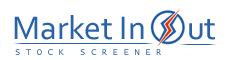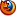Please enable JavaScript to view this page content properly Log In | Sign Up
 Option Analysis
OPTION ANALYSIS

Overview

The most widely used option pricing model is the Black-Scholes option valuation model which was developed by Fisher Black and Myron Scholes in 1973.

The Black-Scholes model helps determine the fair market value of an option based on the security's price and volatility, time until expiration, and the current market interest rate. The following assumptions were made by Black and Scholes when the model was developed:

1. Markets are "frictionless." In other words, there are no transaction costs or taxes; all market participants may borrow and lend at the "riskless" rate of interest; there are no penalties for short selling; and all securities are infinitely divisible (i.e., fractional shares can be purchased).

2. Stock prices are lognormally distributed (i.e., they follow a bell curve). This means that a stock can double in price as easily as it can drop to half its price.

3. Stocks do not pay dividends or make any distributions. (The model is often modified to allow for dividend adjustments.)

4. The option can only be exercised on the expiration date.

The components of the option model are security price, volatility, option life, market interest rate, and dividend (if any).

I suggest you refer to the book Option Volatility and Pricing Strategies, by Sheldon Natenberg for more information on calculations and strategies using the Black-Scholes model.

Interpretation

Put/Call Price

The Put/Call Price is the main output of the Black-Scholes model. It shows how much an option should sell for based on the various components that make up the model (e.g., volatility, option life, security price, etc). It helps answer the question, "Is the option overpriced or underpriced?"

The usefulness of the Put/Call Price is basically two-fold:

1. It helps you locate mispriced options. The option purchaser can use the model to find options that are underpriced. The option writer can use the model to find options that are overpriced.

2. It helps you form a riskless hedge to earn arbitrage profits. For example, you could buy undervalued calls and then short the underlying stock. This creates a riskless hedge because regardless of whether the security's price goes up or down, the two positions will exactly offset each other. You would then wait for the option to return to its fair market value to earn arbitrage profits.

Delta (below) is used to determine the number of shares to purchase in order to form a riskless hedge.

Delta

Delta shows the amount that the option's price will change if the underlying security's price changes by \$1.00.

For example, if XYZ is selling for \$25.00/share, a call option on XYZ is selling for \$2.00 and the Delta is 75%, then the option's price should increase \$0.75 (to \$2.75) if the price of XYZ increases to \$26.00/share. In other words, the option should go up \$0.75 for each \$1.00 that XYZ goes up.

If an option is deep in-the-money, then it will have a high Delta, because almost all of the gain/loss in the security will be reflected in the option price. Conversely, deep out-of-the-money options will have a low Delta, because very little of the gain/loss in the security is reflected in the option price.

If you don't have a computer, the rough rule-of-thumb for calculating Delta is: 75% for an option \$5.00 in the money, 50% for an option at the money, and %25 for an option \$5.00 out of the money.

As an in-the-money option nears expiration, the Delta will approach 100% because the amount of time remaining for the option to move out-of-the-money is small.

Delta is also used to determine the correct number of shares to buy/short to form a "riskless hedge." For example, suppose the Delta on a put option is 66%. A riskless hedge would result from owning a ratio of two-thirds (66%) a position in stock (i.e., 66 shares) to every one long position in a put option contract. If the stock price goes up one point, then the stock position will increase \$66.00. This \$66.00 increase should be exactly offset by a \$66.00 decrease in the value of the put option contract.

As discussed on earlier, forming a riskless hedge gives the investor the potential of earning arbitrage profits, by profiting from the undervalued option's return to its fair market value (i.e., the price at which the option is neither overpriced nor underpriced). Theoretically, the market will eventually value underpriced options at their fair market value . However, it should be noted that high transaction costs may undermine this theory.

Gamma

Gamma shows the anticipated change in Delta, given a one point increase in the underlying security. Thus, it shows how responsive Delta is to a change in the underlying security's price. For example, a Gamma of four indicates that the Delta will increase four points (e.g., from 50% to 54%) for each one point increase in the underlying security's price.

Gamma indicates the amount of risk involved with an option position. A large Gamma indicates higher risk, because the value of the option can change more quickly. However, a trader may desire higher risk depending on the strategy employed.

Option Life

Option Life shows the number of days until expiration. Generally speaking, the longer the time until expiration, the more valuable the option.

A graph of Option Life appears as a stepped line from the upper-left to the lower-right side of the screen. The reason the line is stepped is because of weekends and holidays. For example, on Friday there may be 146 days to expiration and on the following Monday only 143 days remaining.

Theta

Theta shows the change in the option's price (in points) due to the effect of time alone. The longer the time until expiration, the less effect that time has on the price of the option. However, as the option nears expiration, the effect can be great, particularly on out-of-the-money options. Theta is also referred to as "time decay."

For example, a Theta value of -0.0025 means that the option lost 1/4 of one cent due to the passage of time alone.

The effect of time on the option price is almost always positive. The more time until expiration the better chance the option has of being in-the-money at expiration. The only exception to this positive relationship is deep in-the-money put options with an expiration date far into the future.

All other things being equal, options with low Thetas are more preferable (for purchase) than are those with high Thetas.

Vega

Vega shows the change in the option price due to an assumed 1% increase in the underlying security's volatility. Vega shows the dollar amount of gain that should be expected if the volatility goes up one point (all else being equal).

The effect of volatility on the option price is always positive. The greater the volatility of the underlying security, the better chance the option has of being in-the-money at expiration. Therefore, options with higher volatilities will cost more than those with lower volatilities.

Since Vega measures the sensitivity of an option to a change in volatility, options with higher Vegas are more preferable (for purchase) than those with low Vegas.

Volatility

Volatility is a measurement that shows the degree of fluctuation that a security experiences over a given time frame. Wide price movements over a short time frame are characteristic of high volatility stocks.

Volatility is the only input parameter of the Black-Scholes model (e.g., security price, volatility, option life, market interest rate) that is calculated, yet the accuracy of the model is highly dependent on a good Volatility figure. The best measurement of volatility is the one that captures future price movements. But if we knew what future price movements would be, we would care less about the Black-Scholes model--we'd be trading! However, reality forces us to estimate volatility. There are two ways to estimate volatility for use with the Black-Scholes model: Historical Volatility and Implied Volatility.

Historical Volatility measures the actual volatility of the security's prices using a standard deviation based formula. It shows how volatile prices have been over the last x-time periods. The advantage of histocial volatility is that can be calculated using only historical security prices. When you calculate the Black-Scholes put/call price using historial volatililty, most options appear overpriced.

A more widely used measure of option volatility is called Implied Volatility. Implied Volatility is the amount of volatility that the option market is assuming (i.e., implying) for the option. To calculate implied volatility, the actual option price, security price, strike price, and the option expiration date are plugged into the Black-Scholes formula. The formula then solves for the implied volatility.

Options of high volatility stocks are worth more (i.e., carry higher premiums) than those with low volatility, because of the greater chance the option has of moving in-the-money by expiration. Option purchasers normally prefer options with high volatilities and option writers normally prefer options with low volatilities (all else being equal).

Calculation

For exact mathematical formulas used in calculating option values, I recommend Option Volatility and Pricing Strategies by Sheldon Natenberg.

The formula for the Black-Scholes option pricing model is widely available in many books and publications. The original work by Black and Scholes was only done on equity call options. Since their work was originally published, extensions of their model have been developed, such as models for put options and options on futures. Gamma, Theta, and Vega calculations are all extensions of the original Black-Scholes model.

Adjusting the model for dividends provides a more accurate calculation of the option's fair market value. A popular adjustment method assumes that dividend payments are paid out continuously.

 Best viewed in